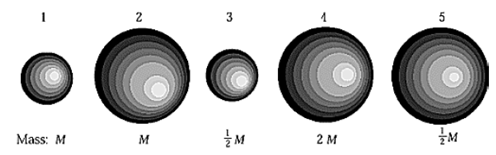# Problem: Five homogeneous planets have relative masses and sizes as shown in the figure. A body of mass m would weigh least on which planet? A. 1 B. 2 C. 3 D. 4 E. 5

###### FREE Expert Solution
94% (123 ratings)
###### Problem Details

Five homogeneous planets have relative masses and sizes as shown in the figure. A body of mass m would weigh least on which planet?

A. 1

B. 2

C. 3

D. 4

E. 5Frequently Asked Questions

What scientific concept do you need to know in order to solve this problem?

Our tutors have indicated that to solve this problem you will need to apply the Acceleration Due to Gravity concept. You can view video lessons to learn Acceleration Due to Gravity. Or if you need more Acceleration Due to Gravity practice, you can also practice Acceleration Due to Gravity practice problems.

How long does this problem take to solve?

Our expert Physics tutor, Jeffery took 2 minutes and 29 seconds to solve this problem. You can follow their steps in the video explanation above.